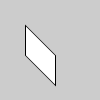# shear_y()#

Shears a shape around the y-axis the amount specified by the angle parameter.

## Examples#def setup():
py5.translate(py5.width/4, py5.height/4)
py5.shear_y(py5.PI/4.0)
py5.rect(0, 0, 30, 30)


## Description#

Shears a shape around the y-axis the amount specified by the angle parameter. Angles should be specified in radians (values from 0 to TWO_PI) or converted to radians with the radians() function. Objects are always sheared around their relative position to the origin and positive numbers shear objects in a clockwise direction. Transformations apply to everything that happens after and subsequent calls to the function accumulates the effect. For example, calling shear_y(PI/2) and then shear_y(PI/2) is the same as shear_y(PI). If shear_y() is called within the draw(), the transformation is reset when the loop begins again.

Technically, shear_y() multiplies the current transformation matrix by a rotation matrix. This function can be further controlled by the push_matrix() and pop_matrix() functions.

Underlying Processing method: shearY

## Signatures#

shear_y(
angle: float,  # angle of shear specified in radians
/,
) -> None


Updated on March 06, 2023 02:49:26am UTC